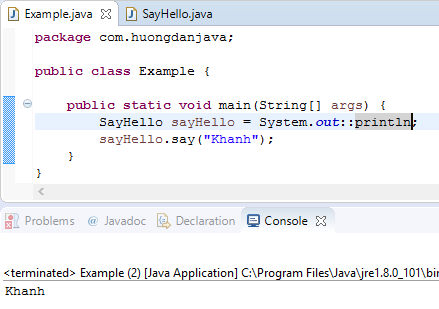# Method reference in Java

Method reference is a new Java concept from version 8. It comes with Lambda Expression, which makes code writing using Lambda Expression even short but still shorter in some cases. In this tutorial, let’s learn more about the method reference in Java!

OK, let’s get started …

First of all, you need to know that the method reference is a more concise way of code for Lambda Expressions which in its body, there is only one method called.

The general syntax for method reference is:

Where Object is the class name containing the method being called (methodName) in the body of the Lambda Expression.

For example, suppose you have a Lambda Expression like this:

In this Lambda Expression, the body part only calls the println() method of the System.out (PrintStream) object. Therefore, we can rewrite this code using the following method reference:

Result:Here, we give all three types of method references.

The first is method reference for a static method:

Suppose I have the following Lambda Expressions:

When moving to the method reference, I can rewrite the following:

The second is method reference for an instance method of an object with a specific data type.

This type can be applied to Lambda Expressions that resemble the following:

Inside, obj is an instance of an object and this obj calls an instance method that uses the remaining arguments in the Lambda Expression expression.

For example, we have the Lambda Expression as follows:

In this expression, we have an argument with the String data type and in the body we use s to call a method that uses the i argument. In this case, we can use the method reference as follows:

The last type is the method reference for an existing object.

With this type, we apply the following Lambda Expressions:

In this form, obj is an instance of an object that has been declared somewhere, obj calls a method that uses an argument in the Lambda Expression expression.

In the example at the top of the tutorial:

println() is an instance method of the PrintStream class. So we can code it as follows: